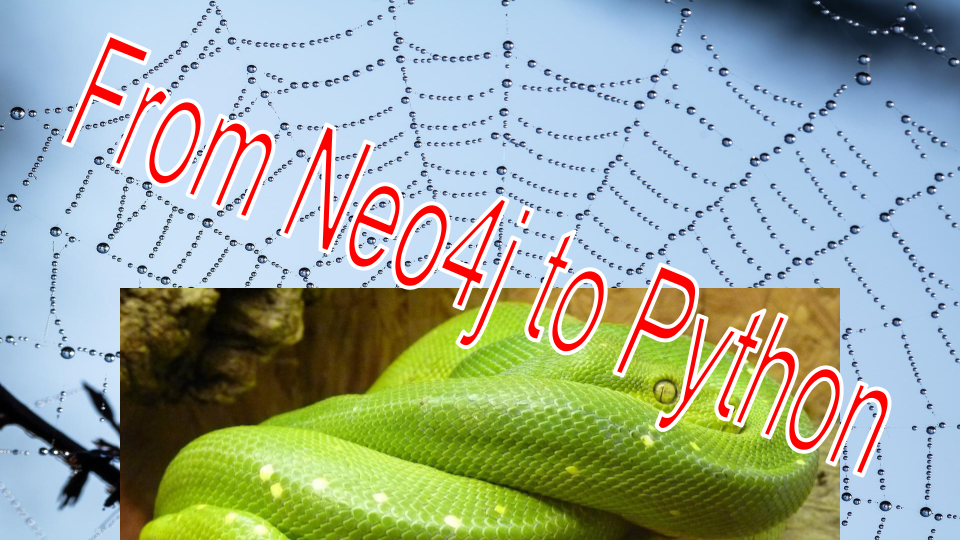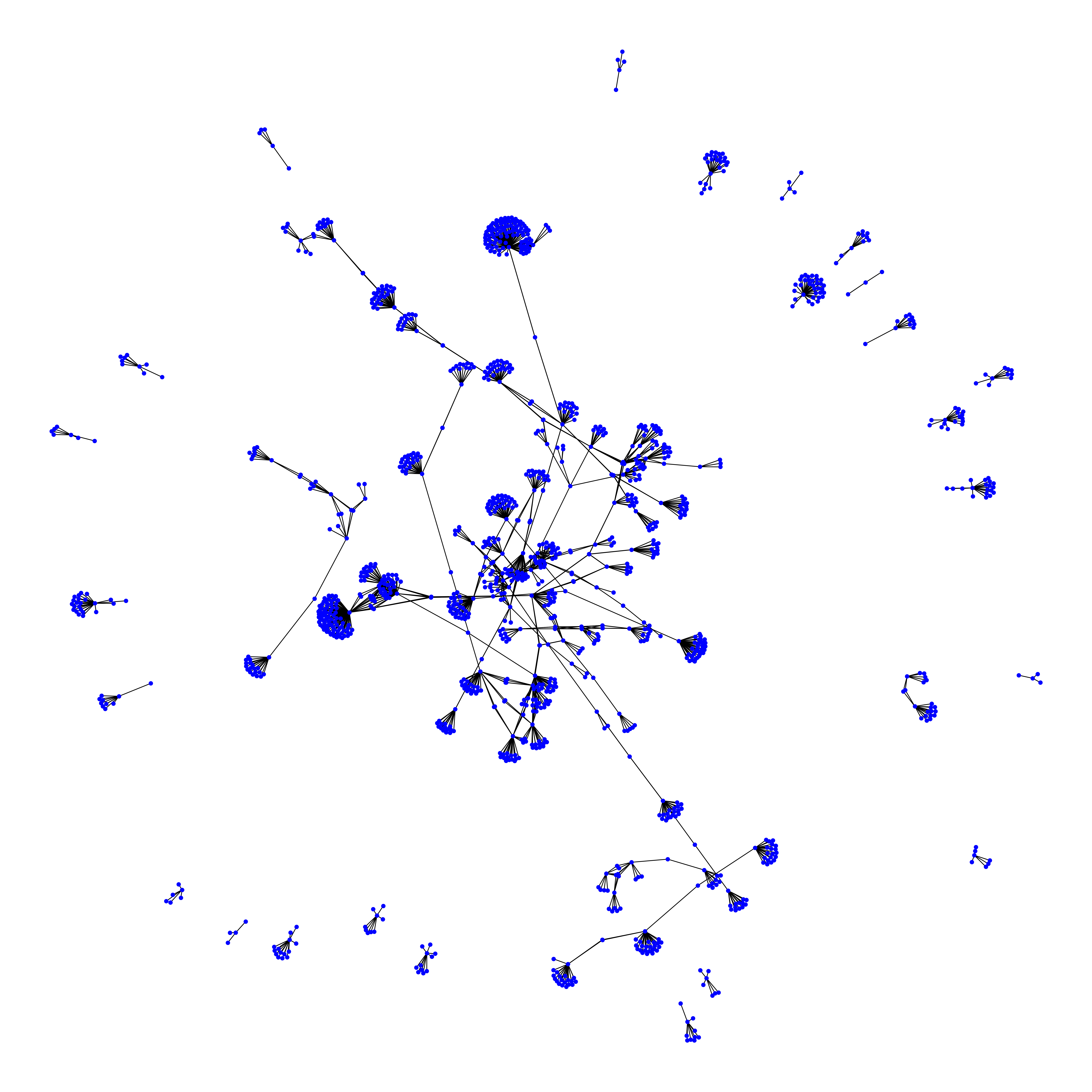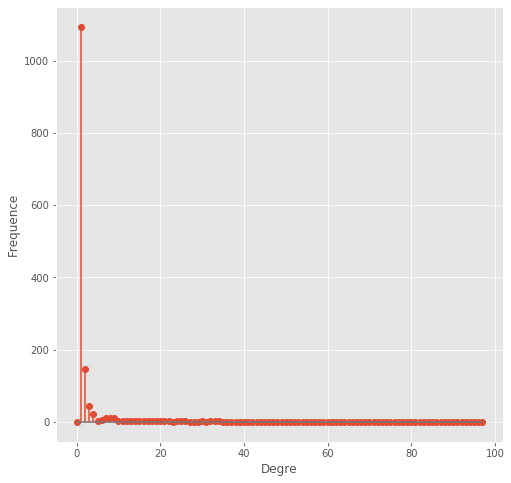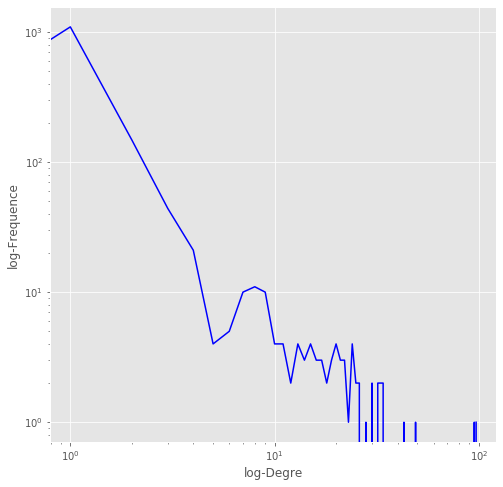Published on 25 Apr 2021 in Data Science

# How to transfer a Neo4j graph to a Python graphNeo4j contains libraries such as GDS and APOC which allow the use of already existing advanced methods and algorithms. But if that’s not enough, in this jupyter notebook, I want to show that we can get the results of Cypher queries on a Python environment and using specialized graph libraries such as networkx we can use advanced algorithms, make measurements and machine learning over graphs.

! pip install neo4j

! pip install py2neo

from neo4j import GraphDatabase

uri = "neo4j://localhost:7687"

connect = GraphDatabase.driver(uri, auth=("neo4j", "************"))

session = connect.session()

query = "match (n)-[p]->(m), (m)-[r]->(j) return *"

results = session.run(query)

import networkx as nx

G = nx.Graph()

nodes = list(results.graph()._nodes.values())
for node in nodes:

rels = list(results.graph()._relationships.values())
for rel in rels:

import matplotlib.pyplot as plt
%matplotlib inline
plt.style.use('ggplot')

options = {
'node_color': 'blue',
'node_size': 800,
'width': 5,

}
plt.figure(figsize = (100,100))
nx.draw(G, with_labels = False, **options)
plt.show()print(nx.info(G))

Name:
Type: Graph
Number of nodes: 1411
Number of edges: 1679
Average degree:   2.3799

degree_freq = nx.degree_histogram(G)
y = degree_freq
plt.figure(figsize=(8, 8))
plt.stem(y)
plt.ylabel("Frequence")
plt.xlabel("Degre")plt.figure(figsize=(8, 8))
plt.loglog(y, 'b-')
plt.ylabel("log-Frequence")
plt.xlabel("log-Degre")degrees = sorted(G.degree, key=lambda x: x, reverse=True)
print(f'Maximum degree: {degrees}')
print(f'Minimum degree: {degrees[-1]}')

Maximum degree: 97
Minimum degree: 1

def get_second_elem(iterable):
return iterable

all_deg = sorted(nx.degree_centrality(G).items(),key=get_second_elem, reverse=True)
print("The 10 nodes with the greatest degree_centrality:")
for node in all_deg[0:10]:
print(node)

The 10 nodes with the greatest degree_centrality:
(4180, 0.06879432624113475)
(4197, 0.0673758865248227)
(4206, 0.03475177304964539)
(4190, 0.030496453900709222)
(4145, 0.024113475177304965)
(4175, 0.024113475177304965)
(4152, 0.02340425531914894)
(4198, 0.02340425531914894)
(4140, 0.02269503546099291)
(4199, 0.02269503546099291)

for d in all_deg:
found_first_author_max_degree = False
for node in nodes:
if (node._labels == {'author'}) and (node._id == d):
print(f"The author {node._id} has the max degree centrality {d}")
print("His name: ",node._properties['name'])
print("His topic: ",node._properties['topic'])
found_first_author_max_degree = True
break
if found_first_author_max_degree:
break


The author 4001 has the max degree centrality 0.005673758865248227
His name:   Attwood, Kristopher M
His topic:  sarcopenia